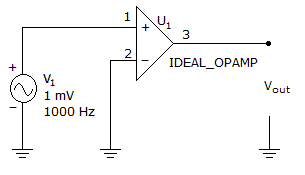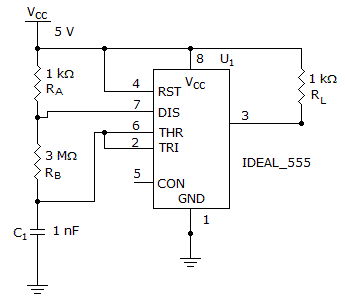# Electronics - Operational Amplifiers

### Exercise :: Operational Amplifiers - General Questions

26.

The voltage follower has a:

 A. closed-loop voltage gain of unity B. small open-loop voltage gain C. closed-loop bandwidth of zero D. large closed-loop output impedance

Explanation:

No answer description available for this question. Let us discuss.

27.

What is the output waveform of the circuit?A. sine wave B. square wave C. sawtooth wave D. triangle wave

Explanation:

No answer description available for this question. Let us discuss.

28.

The ratio between differential gain and common-mode gain is called:

 A. amplitude B. differential-mode rejection C. common-mode rejection D. phase

Explanation:

No answer description available for this question. Let us discuss.

29.

What is the frequency of this 555 astable multivibrator?A. 278 Hz B. 178 Hz C. 78 Hz D. 8 Hz

Explanation:

No answer description available for this question. Let us discuss.

30.

If the gain of a closed-loop inverting amplifier is 3.9, with an input resistor value of 1.6 kilohms, what value of feedback resistor is necessary?

 A. 6240 ohms B. 2.4 kilohms C. 410 ohms D. 0.62 kilohms